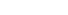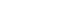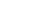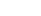How Cheenta works to ensure student success?
Explore the Back-Story

# Test of Mathematics Solution Subjective 181 - Diagonal MovesThis is a Test of Mathematics Solution Subjective 181 (from ISI Entrance). The book, Test of Mathematics at 10+2 Level is Published by East West Press. This problem book is indispensable for the preparation of I.S.I. B.Stat and B.Math Entrance.

Also visit: I.S.I. & C.M.I. Entrance Course of Cheenta

## Problem

Suppose that one moves along the points (m, n ) in the plane where m and n are integers in such a way that each move is a diagonal step, that is, consists of one unit to the right or left followed by one unit either up or down.

(a) Which point (p, q) can be reached from the origin.

(b) What is the minimum number of moves needed to reach such a point (p, q)?

## Discussion

For each horizontal movement (right or left) there is one vertical movement (up or down). Suppose there are R right moves, L left moves. Also there be U upward move and D downward move. Since number of horizontal movement equals number of vertical movements hence we have

R+L = U+D = k (say)

The final coordinate reached is (R - L , U - D) (as each right move counts as +1 and left move counts as -1, and similarly for vertical movements),

L = k -R

D = k -U

Hence the final coordinate is (2R - k, 2U - k)

Therefore both the coordinates are of same parity (that is either both are even or both are odd).

So we have shown that the final point that can be reached has both x and y coordinate of the same parity. Now we show the converse. That is all the points whose x and y coordinates are of same parity can be reached.

Say P be a point whose both coordinates are even. Without loss of generality we may say P = (2m, 2n) whereand both coordinates are positive.

1. 2(m-n) moves of the form Right-Up followed by Left-down is performed to reach the point (2(m-n) , 0)

2. Next 2n moves of the form Right-Up is performed to reach the point (2m, 2n)

So in total 2(m-n) + 2n = 2m moves are performed to reach the point (2m, 2n)

Similarly to reach a point whose both coordinates are odd, say (2j+1, 2l + 1),  we first reach the point (2j, 2l) and then move one more unit diagonally upward.

This same algorithm will work for all the quadrants.

Also if (p, q) is the final coordinate with p > q, we have taken p steps to reach the point. It is not possible to reach the point in less than p steps, because we must cover at least p boxes in at least one direction and in one step exactly one box can be covered.

Therefore we have found the answer:

(1) All the points (p, q) can be reached where(2) If, minimum number of steps required is p. If, the minimum number of steps required is q.This is a Test of Mathematics Solution Subjective 181 (from ISI Entrance). The book, Test of Mathematics at 10+2 Level is Published by East West Press. This problem book is indispensable for the preparation of I.S.I. B.Stat and B.Math Entrance.

Also visit: I.S.I. & C.M.I. Entrance Course of Cheenta

## Problem

Suppose that one moves along the points (m, n ) in the plane where m and n are integers in such a way that each move is a diagonal step, that is, consists of one unit to the right or left followed by one unit either up or down.

(a) Which point (p, q) can be reached from the origin.

(b) What is the minimum number of moves needed to reach such a point (p, q)?

## Discussion

For each horizontal movement (right or left) there is one vertical movement (up or down). Suppose there are R right moves, L left moves. Also there be U upward move and D downward move. Since number of horizontal movement equals number of vertical movements hence we have

R+L = U+D = k (say)

The final coordinate reached is (R - L , U - D) (as each right move counts as +1 and left move counts as -1, and similarly for vertical movements),

L = k -R

D = k -U

Hence the final coordinate is (2R - k, 2U - k)

Therefore both the coordinates are of same parity (that is either both are even or both are odd).

So we have shown that the final point that can be reached has both x and y coordinate of the same parity. Now we show the converse. That is all the points whose x and y coordinates are of same parity can be reached.

Say P be a point whose both coordinates are even. Without loss of generality we may say P = (2m, 2n) whereand both coordinates are positive.

1. 2(m-n) moves of the form Right-Up followed by Left-down is performed to reach the point (2(m-n) , 0)

2. Next 2n moves of the form Right-Up is performed to reach the point (2m, 2n)

So in total 2(m-n) + 2n = 2m moves are performed to reach the point (2m, 2n)

Similarly to reach a point whose both coordinates are odd, say (2j+1, 2l + 1),  we first reach the point (2j, 2l) and then move one more unit diagonally upward.

This same algorithm will work for all the quadrants.

Also if (p, q) is the final coordinate with p > q, we have taken p steps to reach the point. It is not possible to reach the point in less than p steps, because we must cover at least p boxes in at least one direction and in one step exactly one box can be covered.

Therefore we have found the answer:

(1) All the points (p, q) can be reached where(2) If, minimum number of steps required is p. If, the minimum number of steps required is q.

### Knowledge Partner